ISEE Lower Level Math : Probability

Example Questions

← Previous 1 3 4 5 6 7 8 9 10

Example Question #1 : Outcomes

If Jennifer has a bag of hair ties that containsblue hair tie,green hair ties,pink hair ties, andpurple hair tie, and she grabs one at random, what is the probability she will grab a green hair tie?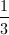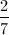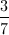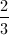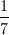Explanation:

First we must determine how many hair ties are in the bag by adding all of them together.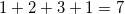We know from the information provided in the problem that there aregreen hair ties in the bag, so the probability of Jennifer grabbing one green hair tie from the seven possible hair ties is.

Example Question #1 : Probability

Express the probability as a percent, rounded to the nearest whole number.

Matt had a bag of 28 colored candies.  If he had 6 red, 5 purple, 7 orange, and 10 yellow, what is the probability that he pulls out a red-colored candy?

21%

6%

30%

18%

25%

21%

Explanation:

Since there are 6 reds out of 28, divide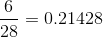.

Move the decimal 2 places to the right 21.4% and round to the nearest whole number21%.

Example Question #1 : Outcomes

If Brian has a box of toy cars that containsblue cars,red cars,white cars andyellow car, what is the probability that he will pick up a white car?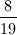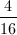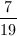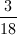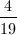Explanation:

First we must determine how many toy cars Brian has in the box by adding them together.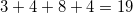We know from the information provided that there arewhite cars in the box, so the probability of Brian picking up one white car from thepossible cars is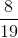.

Example Question #1 : Probability

Determine the probability of the outcome. Express the probability as a percent, rounded to the nearest whole number.

Erin saw a basket full of tiny bouncy balls. There were 27 green balls, 13 red balls, 11 yellow balls, and 13 spotted balls. What is the probability that Erin will choose a spotted ball or a yellow ball?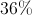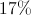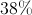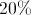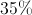Explanation:

To find the probability of an outcome, set up a fraction: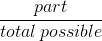.

Since there are 13 yellow balls PLUS 11 yellow balls (part) out of 64 balls in all (total possible) the fraction we can find what the fraction looks like.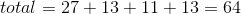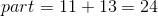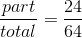If you reduce the fraction by 8 (because the numerator and the denominator are both divisible by 8), it can be simplified.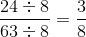Since the question asks you to state the probability as a percent, we need to convert fraction to percent. We can set up a proportion.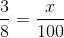Cross multiply and solve for.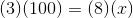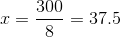This equals 37.5. Rounded to the nearest whole number gives us 38%.

Example Question #1 : Probability

A card is chosen at random from a standard deck of fifty-two cards. What is the probability that the card will be a queen or a heart, but not both?.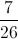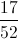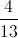.

Explanation:

Out of fifty-two cards, there are thirteen hearts, but since we are excluding the queen, this makes twelve cards that we are including. Out of the four queens, we include three of them - again, excluding the queen of hearts. This makes fifteen cards out of fifty-two, so the probability of drawing one of them is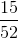.

Example Question #6 : Probability

A card is chosen at random from a standard deck of fifty-two cards. What is the probability that the card will be a king, a spade, or both?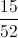Explanation:

Out of fifty-two cards, there are thirteen spades; also, there are four kings, but one is a spade, so this makes three additional cards for a total of sixteen. The probability of drawing a card that is a king and/or a spade is therefore sixteen out of fifty-two, or: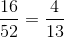Example Question #1 : Probability

A bag contains one token for every multiple of 6 from 1-90 (inclusive). If you draw out one token at random, what are the odds of it being a multiple of 4?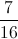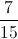Explanation:

The simplest way to solve this is to make a chart of all of the multiples of 6 (just count by 6) from 1-90:

6     12     18     24     30

36    42     48     54     60

66    72     78     84     90

That makes 15 numbers, which will be our denominator.

Next, look through the list and identify the numbers that are divisible by 4 (divisible by 2, and then 2 again).

6     12     18     24     30

36    42     48     54     60

66    72     78     84     90

That is 7 numbers. Thus,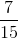of these multiples of 6 are also divisible by 4.

Example Question #8 : Probability

Susan has a bag of 12 assorted candies that included 3 blue, 2 green, 5 red, and 2 pink. If Susan picks one piece of candy without looking, what are the chances that she picks a blue candy?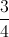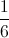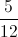Explanation:

To fine the chance of Susan picking a blue candy, consider how many blue candies there are: 3

Compared to how many candies there are total: 12

That means the chance of Susan picking a blue candy is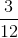, which simplifies to.

Example Question #9 : Probability

Marcus has a box of 24 crayons. 8 are blue, 6 are green, 4 are red, 3 are black, 2 are white, and 1 is purple. If he randomly picks a crayon from the box, which color has a 1 out of 6 chance of being chosen?

black

green

red

blue

red

Explanation:

Red here is the correct answer. Marcus has a 4 out of 24 chance of choosing red, as there are 4 red crayons in a box of 24. Reduce the probability, and you get 1 out of 6.

Example Question #1 : Probability

Jessica has a pencil box with her favorite pencils and erasers.  In the box there are different colored erasers.  There are 4 pink, 3 blue, 10 yellow and 4 red erasers.  What is the probability that Jessica will select an eraser from the box at random that is NOT red?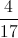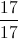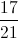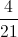Explanation:

To find the probability of Jessica NOT picking a red eraser is the same as finding the probability that it is everything else that she wants.

First find the total number of erasers Jessica has by adding all of them no matter what the color may be.  These are all the options whether she wanted them or not.  In total there are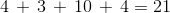total erasers.

Then count all of the erasers that are NOT red.  That would be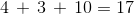erasers that are NOT red.

The probability would be set up as: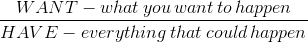So, you would have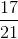← Previous 1 3 4 5 6 7 8 9 10